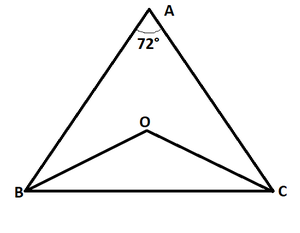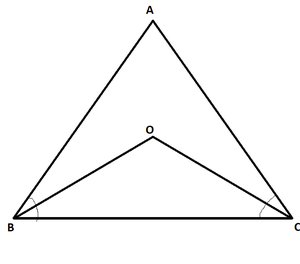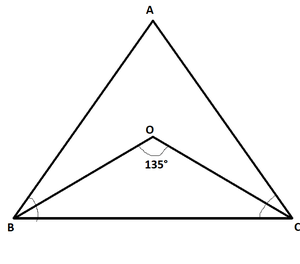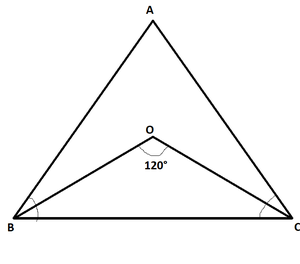Open In App
Related Articles
• RD Sharma Class 9 Solutions

# Class 9 RD Sharma Solutions – Chapter 9 Triangles and its Angles- Exercise 9.1

### Question 1: In a triangle ΔABC, if ∠A = 55° ∠B = 40°, find ∠C.

Solution:

Given: A = 55° and B = 40°

Theorem Used: Sum of all angles of a triangle is 180°.

From the theorem we can write that:

∠A + ∠B + ∠C = 180°

55° + 40° + ∠C = 180° //Putting the values of A and B.

95° + ∠C = 180°

∠C = 180° – 95°

∠C = 85°

The angle ∠C is 85°.

### Question 2: If the angles of a triangle are in the ratio 1:2:3, determine three angles.

Solution:

Given: Angles of a triangle are in the ratio 1:2:3

Let the angles be x, 2x, 3x

Theorem Used: Sum of all angles of a triangle is 180°.

x + 2x + 3x = 180°

6x = 180°

x = 180°/6

x = 30°   //Deriving the value of x

Deriving the value of the other two angles from the value of x

2x = 2 X (30°) = 60°

3x = 3 X (30°) = 90°

All the three angles are 30°,60° and 90° respectively.

### Question 3: The angles of a triangle are (x − 40)°, (x − 20)° and (1/2 x − 10)°. Find the value of x.

Solution:

The angles of a triangle are (x − 40)°, (x − 20)° and (1/2x − 10)°

Theorem Used: Sum of all angles of a triangle is 180°.

(x − 40)° + (x − 20) ° + (1/2 x − 10)° = 180°

5/2 x – 70° = 180°

5/2 x = 180° + 70°

5x = 2(250)°

x = 500°/5

x = 100°

The value of x is 100°

### Question 4: The angles of a triangle are arranged in ascending order of magnitude. If the difference between two consecutive angles is 10°, find the three angles.

Solution:

Given: The difference between two consecutive angles is 10°.

Theorem Used: Sum of all angles of a triangle is 180°.

Let the smallest angle of the triangle is x°.

Hence, according to the given condition, the other two consecutive angles are (x + 10)° and (x + 20)° respectively.

Now from the theorem mentioned we can write that:

x + (x + 10°) + (x + 20°) = 180°.

3x + 30° = 180° //Simplifying the equation

3x = 180° – 30°

3x = 150°

x = 150°/3

x = 50°

Hence, here we get the smallest angle is 50°.

The next consecutive angles are, 50° + 10° = 60° and 50° + 20° = 70° respectively.

Hence, the three angles of the triangle are 50°, 60°, and 70° respectively.

### Question 5: Two angles of a triangle are equal and the third angle is greater than each of those angles by 30°. Determine all the angles of the triangle.

Solution:

Given: (i) Two angles of a triangle are equal

(ii)The third angle is greater than each of those angles by 30°

Theorem Used: Sum of all angles of a triangle is 180°.

Let the equal angles are x° and the other angle is (x+30)°.

Now from the theorem mentioned we can write that:

x + x + (x + 30°) = 180°

3x + 30° = 180°

3x = 180° – 30°

3x = 150°

x = 150°/3

x = 50°

Hence, the equal angles are 50° each and the other angle is (50 + 30)° = 80°.

The angles of the triangle are 50°, 50° and 80° respectively.

### Question 6: If one angle of a triangle is equal to the sum of the other two, show that the triangle is a right-angle triangle.

Solution:

Given: one angle of a triangle is equal to the sum of the other two

Theorem Used: Sum of all angles of a triangle is 180°.

Let the three angles of the triangle are ∠A, ∠B and ∠(A+B) respectively.

∠A + ∠B + ∠(A + B) = 180°

2(∠A + ∠B) = 180°

∠A + ∠B = 90°  //Hence the third angle A + B = 90° (Proved)

### Question 7: ABC is a triangle in which angle ∠A = 72°. The internal bisector of angle ∠B and ∠C meet in O. Find the magnitude of ∠BOC.

Solution:

Given: (i) ∠A = 72° of the triangle ABC

(ii)Internal bisectors of angle ∠B and ∠C meet in point O.Theorems Used: Sum of all three angles of a triangle is 180°

In triangle ABC,

∠A + ∠B + ∠C = 180°

72° + ∠B + ∠C = 180°

∠B + ∠C = 180° – 72° = 108°

∠B/2 + ∠C/2 = 108°/2 = 54°                         // Dividing both sides by 2

∠OBC + ∠OCB = 54°  –derivation (1)           // From the triangle we can clearly see this as OB and OC are the angle bisectors

Now in △BOC,

∠OBC + ∠OCB + ∠BOC = 180°

∠BOC + (∠OBC + ∠OCB) = 180°

∠BOC + 54° = 180°    //Putting the value of ∠OBC + ∠OCB = 54° from derivation(1)

∠BOC = 180° – 54° = 126° (Ans)

∠BOC = 126°.

### Question 8: The bisectors of the base angles of a triangle cannot enclose a right angle at any case.

Solution:Theorems Used: Sum of all three angles of a triangle is 180°

From the triangle △ABC,

∠A + ∠B + ∠C = 180°

∠A/2 + ∠B /2 + ∠C/2 = 180°/2 = 90°   //Dividing both sides by 2

∠B /2 + ∠C/2 = 90° – ∠A/2  —-(1)

From the triangle △BOC,

∠BOC + ∠OBC + ∠OCB = 180°

As OB and OC are the angle bisectors, ∠OBC = ∠B/2 and ∠OCB = ∠C/2.

∠BOC + ∠B /2+ ∠C/2 = 180°          //Putting the values of ∠B /2+ ∠C/2  = 90° – ∠A/2     from the previous derivation,

∠BOC + 90° – ∠A/2 = 180°

∠BOC = 180° – 90° + ∠A/2 = 90° + ∠A/2

For any valid triangle △ABC ∠A > 0,  It implies that ∠A/2 > 0,

That simply means

∠BOC not equals to 90° at any case. (proved)

### Question 9: If the bisectors of base angles of a triangle enclose an angle of 135°, Prove that the triangle is a right-angle triangle.

Solution:Given: In △BOC the ∠BOC = 135°

Theorems Used: Sum of all three angles of a triangle is 180°

From the triangle △ABC,

∠A + ∠B + ∠C = 180°

∠A/2 + ∠B /2+ ∠C/2 = 180°/2 = 90°   //Dividing both sides by 2

∠B /2+ ∠C/2 = 90° – ∠A/2  —-(1)

From the triangle △BOC,

∠BOC + ∠OBC + ∠OCB = 180°

As OB and OC are the angle bisectors, ∠OBC = ∠B/2 and ∠OCB = ∠C/2.

∠BOC + ∠B/2 + ∠C/2 = 180°          //Putting the values of ∠B/2 + ∠C/2  = 90° – ∠A/2     from the previous derivation,

∠BOC +  90° – ∠A/2 = 180°

∠BOC = 180° – 90° + ∠A/2 = 90° + ∠A/2

Putting the value ∠BOC = 135° from the given condition,

90° + ∠A/2 = 135°

∠A/2 = 135° – 90° = 45°

∠A = 45° X 2 = 90°

Hence, △ABC is a right angle triangle(proved)

### Question 10: In a triangle △ABC, ∠ABC = ∠ACB and the bisector of ∠ABC and ∠ACB intersect at O such that ∠BOC = 120°. Show that ∠A = ∠B = ∠C = 60°.

Solution:Given: (i)∠ABC = ∠ACB

(ii)∠BOC = 120°

From the triangle △ABC,

∠ABC = ∠ACB

∠ABC/2 = ∠ACB/2

∠OBC = ∠OCB

From the triangle △ABC,

∠OBC + ∠OCB + ∠BOC = 180°

From the given condition ∠BOC = 120°, and ∠OBC = ∠OCB

We can write that,

∠OBC + ∠OBC + 120° = 180°.

2 X ∠OBC = 180° – 120° = 60°

∠ABC = 60°

As angle ∠ACB = ∠ABC,

∠ACB = 60°

∠ACB + ∠ABC + ∠BAC = 180°

60° + 60° + ∠BAC = 180°

∠BAC = 180° – 120° = 60°

Hence,

∠A = ∠B = ∠C = 60°.(Proved)

### (i) Two right angles.

If the triangle have two right angles, sum of those angles become 90° + 90° = 180° , That implies that the size of the third angle is 180° – 180° = 0, That is not possible,

### (ii)Two obtuse angles

The size of an obtuse angle is greater than 90° , Hence the sum of both angles are greater than 180° , But we know sum of all three angles of a triangle is 180°. So it is not possible.

### (iii) Two acute angles

Having two acute angles does not violates any law as their  sum is less than 180°

### (iv) All Angles More than 60°

Having all angles more than 60° will make the sum of all angles > 180°.But we know sum of all three angles of a triangle is 180° . So it is not possible.

### (v) All Angles Less than 60°

Having all angles more than 60° will make the sum of all angles < 180°. But we know sum of all three angles of a triangle is 180° . So it is not possible.

### (vi) All angles equal to 60°

Having all angles equal to 60° will make the sum of all angles = 180°.And we know sum of all three angles of a triangle is 180° . So it is possible.

### Question 12: If each angle of a triangle is less than the sum of the other two, Show that all angles of the triangle are acute angle.

Solution:

Given: Each angle is less than the sum of the other two

∠A + ∠B + ∠C = 180°

Given that ,∠A < ∠B + ∠C, So we can write,

∠A < 90°,

The same thing can be done for ∠B and ∠C.

Hence, it is proved that all the three angles are acute angle.